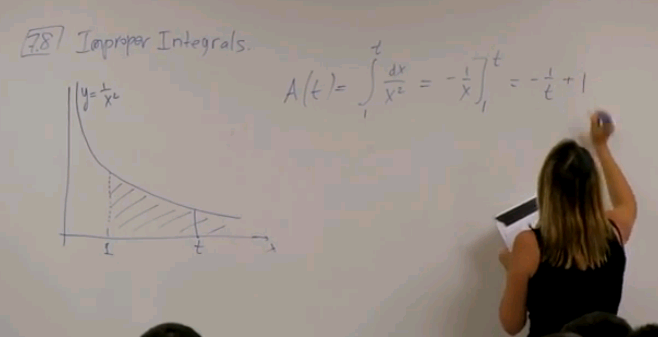# Home

## Math 2B. Lec. 16. Calculus: Improper Integrals (English)

UCI Math 2B: Single-Variable Calculus (Fall 2013)
Lec 16. Single-Variable Calculus -- Improper Integrals --
View the complete course: http://ocw.uci.edu/courses/math_2b_calculus.html
Instructor: Natalia L. Komarova Ph.D.

More courses at http://ocw.uci.edu

Description: UCI Math 2B is the second quarter of Single-Variable Calculus and covers the following topics: Definite integrals; the fundamental theorem of calculus. Applications of integration including finding areas and volumes. Techniques of integration. Infinite sequences and series. Parametric and polar equations.

Recorded on November 4, 2013